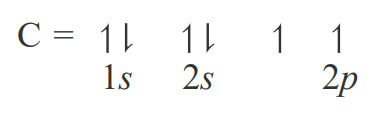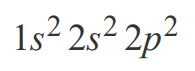# Problem: Part C. The electron configuration can also be represented by writing the symbol for the occupied subshell and adding a superscript to indicate the number of electrons in that subshell. For example, consider a carbon atom having an atomic number of 6. The total number of electrons in a neutral carbon atom is 6. The electron configuration of the carbon atom represented by the orbital diagram isThis electron configuration can be written aswhere 1s, 2s, and 2p are the occupied subshells, and the superscript "2" is the number of electrons in each of these subshells. Use the rules for determining electron configurations to write the electron configuration for P. Express your answer in complete form in order of orbital filling. For example, 1s2 2s2 should be entered as 1s22s2.

###### FREE Expert Solution
99% (64 ratings)View Complete Written Solution
###### Problem Details

Part C. The electron configuration can also be represented by writing the symbol for the occupied subshell and adding a superscript to indicate the number of electrons in that subshell. For example, consider a carbon atom having an atomic number of 6. The total number of electrons in a neutral carbon atom is 6. The electron configuration of the carbon atom represented by the orbital diagram isThis electron configuration can be written aswhere 1s, 2s, and 2p are the occupied subshells, and the superscript "2" is the number of electrons in each of these subshells. Use the rules for determining electron configurations to write the electron configuration for P. Express your answer in complete form in order of orbital filling. For example, 1s2 2s2 should be entered as 1s22s2.